1.

Write the sum using summation notation, assuming the suggested pattern continues.

1 – 3 + 9 – 27 + … (2 points)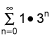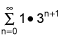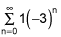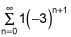2.

Write the sum using summation notation, assuming the suggested pattern continues.

-9 – 4 + 1 + 6 + … + 66 (2 points)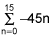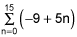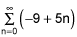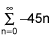3.

Write the sum using summation notation, assuming the suggested pattern continues.

16 + 25 + 36 + 49 + … + n2 + … (2 points)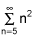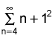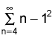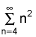4.

Find the sum of the arithmetic sequence.

-10, -7, -4, -1, 2, 5, 8 (1 point)

11

-70

0

-7

5.

Find the sum of the geometric sequence. (1 point)

1,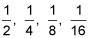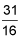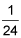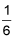93

6.

Find the sum of the first 8 terms of the sequence. Show all work for full credit.

1, -3, -7, -11, … (2 points)